# A physically unrealizable problem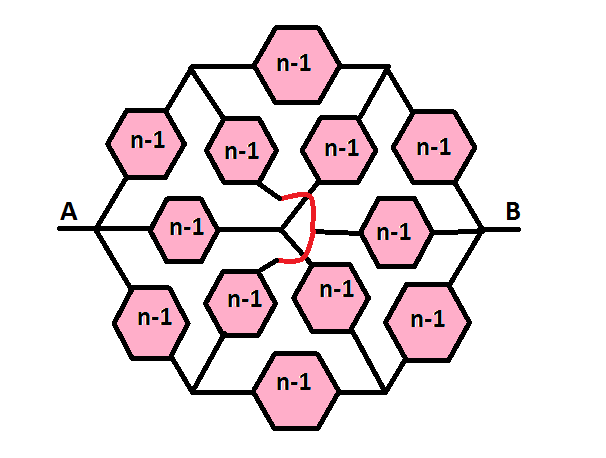Let us define a hexaresistor (depicted below)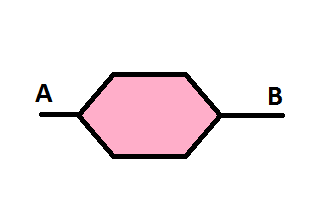as a hexagonal resistive network which can be described as below: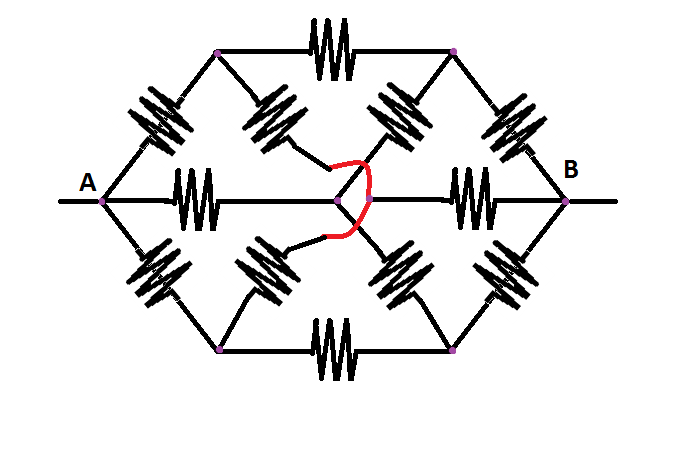with all the resistors having a value of R.

Then, let us define a second order hexaresistor (depicted below)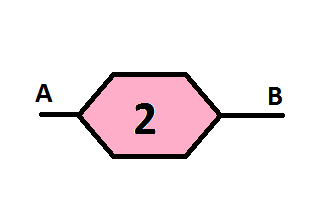as a network which can be described as a hexagonal resistive network of hexaresistors as shown below: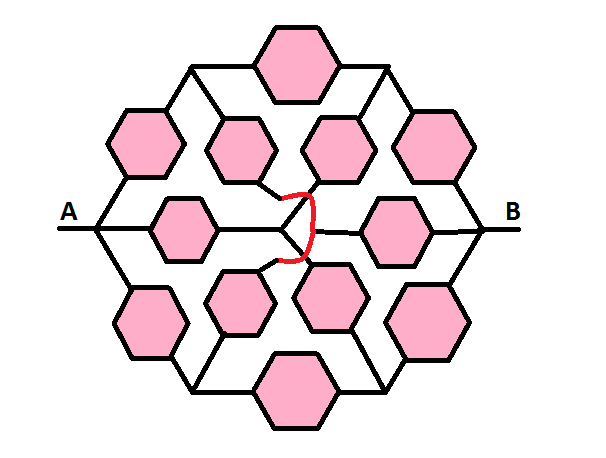If we construct an identical hexagonal resistive network of second order hexaresistors, we will be able to create a third order hexaresistor , and so on, such that an n-order hexaresistor, depicted below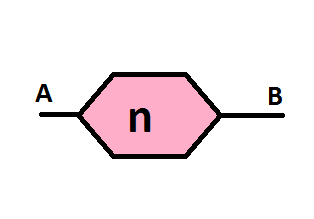is a network of.

If R= 1000 ohms, determine the resistance from points A to B, where B extends to infinity.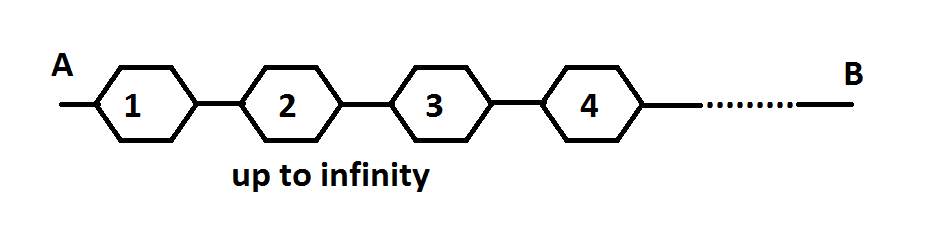Note: This is physically unrealizable, but theoretically, a particular value is approached.

×